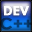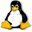Home Latest News Archive Tutorials C++ 2 3 4 Win32 Java OpenGL GLUT OpenGL ES 2 3 4 DirectX VB.NET HTML Photoshop 3dsmax Forums Projects Modelling Links About Us Contact Search Recent Tutorials C / C++ Win32 OpenGL OpenGL ES GLUT## Character Strings

Tutorials > C++ > Character Strings

### Introduction

Almost every program you write will normally involve some kind of character manipulation. This tutorial will explain a number of functions that will make manipulation of C style strings possible. These functions are contained in the stdlib.h header file. The functions we will cover are as follows :

strlen(const char *)
strchr(const char *, char)
strrchr(const char *, char)
strstr(const char *, const char *)
strcmp(const char *, const char *)
stricmp(const char *, const char *)
strcpy(char *, const char *)
strncpy(char *, const char *, int)

Contents of main.cpp :

If you are using Unix / Linux, you may need to include the string.h header file.

```#include <iostream>
#include <stdlib.h>

using namespace std;

int main()
{
```

First we create a character string. Note that we do not use a char *. Instead we use an array of characters. This is because you cannot edit a char *.

```	char str = "Hello World";
```

Below we use the strlen function. This function takes a character string and returns the length of the string. In our case, 11 will be returned.

```	cout << "String Length : ";
cout << (int)strlen(str) << endl;
```

The strcat function allows you to append one string at the end of another. The first parameter is the string that will be changed and the second parameter is the string that you want appended. The code below appends ", Bob!" onto the end of "Hello World".

```	cout << "Appended String : ";
strcat(str, ", Bob!");
cout << str << endl;
```

The strchr function allows you to find an occurrence of a specific character. The first parameter is the string that you will be searching and the second parameter is the character that you are looking for. The memory address of the character if found is returned. If no matching character is found, NULL is returned.

If we pass the character's memory address onto the cout object, the string starting from the ',' is printed out. This is because the cout object prints out characters until it reaches the '\0' terminating character.

```	cout << "String from , : ";
cout << strchr(str, ',') << endl;
```

If we want to find the exact position of the character, we can subtract the memory address specifying the start of the original string from the memory address that is found. The value of 11 is therefore returned in our case.

```	cout << "Position of , : ";
cout << (int)(strchr(str, ',') - str) << endl;
```

The strchr function returns the address of the first occurrence of a specific character. You may want to rather find the last occurrence of a specific character. This can be achieved using the strrchr function. This function operates in the exact same way as the strchr function except that it returns the memory address of the last occurrence of a specific character.

```	cout << "First position of o : ";
cout << (int)(strchr(str, 'o') - str) << endl;
cout << "Last position of o : ";
cout << (int)(strrchr(str, 'o') - str) << endl;
```

Whereas before you were searching for the occurrence of a specific character, you can also search for an entire string of characters. This can be achieved using the strstr function. The first parameter is the string that you are searching through and the second parameter is the substring that you are looking for. The memory address of the string is returned if found otherwise NULL is returned. The code below therefore outputs the value of 6 as the 'W' of "World" starts at position 6 in the string.

```	cout << "World Substring : ";
cout << (int)(strstr(str, "World") - str) << endl;
```

When comparing strings, you cannot simply say for eg. if (str1 == str2) as this would compare memory addresses. The function strcmp is therefore used to compare strings. The function takes two parameters which are the two strings that you are comparing. The value returned is determined as follows :

 Return Value Meaning < 0 The first parameter is less than the second. eg. Albert is less than Grant 0 Both strings are equal. > 0 The first parameter is greater than the second.

Our code below produces a negative value as the first parameter is less than the second. Our message is therefore not displayed.

```	char str1 = "Hello";
char str2 = "hello";

if (!strcmp(str1, str2))
cout << "Strings are the same." << endl;
```

You may want to compare strings while ignoring their case. The stricmp function can be used to achieve this. The function works in the exact same way as strcmp. This results in our code below printing out the message displaying that both strings are the same ignoring case. If you are running Unix / Linux, this function may not be available. Instead you would use the function strcasecmp which works in the exact same way.

```	if (!stricmp(str1, str2))
cout << "Strings are the same "
<< "ignoring case." << endl;
```

You may want to copy the data in one string variable to another. You cannot just use the = operator directly eg. str2 = str1 as this causes str2 to use the same memory address as str1. The strcpy function allows you to effectively copy the contents of one string to another. The first parameter is the place where you would like to store the copied data and the second parameter is the string that you want to copy from. Our code below therefore copies that data from str1 into str2.

```	cout << "String Copy : ";
strcpy(str2, str1);
cout << str2 << endl;
```

You may not want to copy an entire string. You may want to copy only a substring. This can be achieved using the strncpy function. The first parameter specifies the variable where you would like the resulting string to be stored. The second parameter specifies the memory address from where you would like the copy to start and the third parameter specifies how many charcters to copy. Our code below therefore starts copying from the 'W' which is in position 6 and copies 5 characters. This results in the string "World" being copied into the str1 variable.

```	cout << "Substring Copy : ";
strncpy(str1, str + 6, 5);
cout << str1 << endl;

system("pause");

return 0;
}```

You have now been provided with a valuable resource for manipulating C style strings. This information should now help you in all your programs as strings will be used in the majority of your programs.

Please let me know of any comments you may have : Contact Me

 Source Files :< Tutorial 31 - Enumerations Tutorial 33 - C++ Strings >

All Rights Reserved, © Zeus Communication, Multimedia & Development 2004-2005

Read the Disclaimer